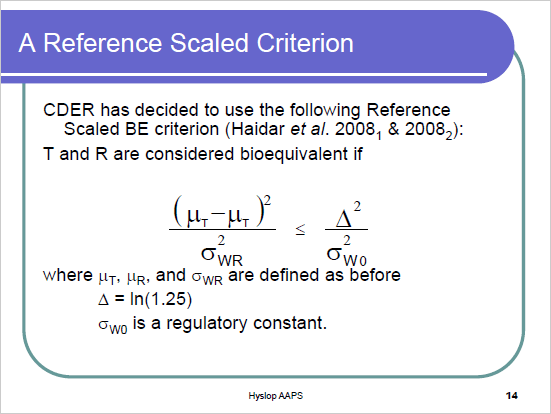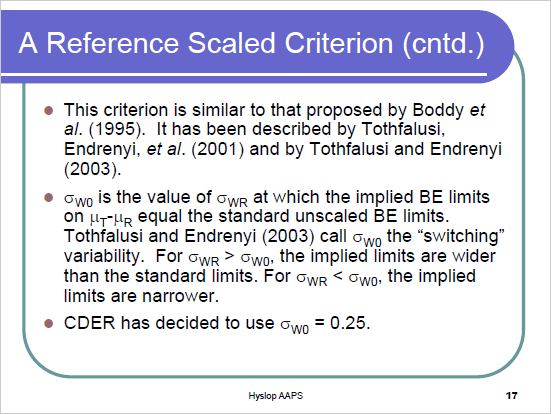## Inflation type one error: FDA [RSABE / ABEL]

Hi Mikalai,

❝ […] what regulators say on this subject? I do not remember any reflection on this matter in official documents (EMA, FDA)?

Some slides of Terry Hyslop (Director, Division of Biostatistics) of his presentation “Bioequivalence (BE) for Highly Variable Drugs” at the AAPS Workshop (New Orleans, Nov 2010) – after the progesterone guidance was published…Aka the ‘implied limits’ (see this post and slide 23 below).Well roared, lion! Trouble starts because we use $$s_{WR}$$ instead of the unknown $$\sigma_{WR}$$.
Nasty but $$s_{WR}$$ is all we have.
… use scaled average BE if sWR > cutoff.Illegible text (white with grey shadowing):
assuming no subject-by-formulation interaction, σWT = σWR,
true GMR = max (1.25, implied scaled BE limit)

What‽
library(PowerTOST) swR           <- sort(c(CV2se(0.3), 0.294, seq(0.2, 0.3, 0.01))) CVwR          <- se2CV(swR) reg           <- reg_const("FDA") reg$CVswitch <- se2CV(0.294) reg$pe_constr <- FALSE # pure RSABE (without PE constraint) GMRs          <- data.frame(GMR       = scABEL(CV = CVwR,                                                regulator = reg)[, "upper"],                             GMR.Terry = exp(reg$r_const * swR)) res <- data.frame(swR = swR, CVwR = CVwR, GMR = GMRs$GMR,                             TIE = NA_real_, GMR.max = NA_real_,                             TIE.Terry = NA_real_) for (j in seq_along(swR)) {   # Cheating: That is not implemented in the guidance!   res$GMR.max[j] <- max(c(1.25, GMRs$GMR.Terry[j]))   res$TIE.Terry[j] <- power.RSABE(CV = CVwR[j], theta0 = res$GMR.max[j],                                   design = "2x3x3", n = 36, nsims = 1e6)   # That is correct!   res$TIE[j] <- power.RSABE(CV = CVwR[j], theta0 = res$GMR[j],                                   design = "2x3x3", n = 36, nsims = 1e6) } reg; print(res, digits = 4, row.names = FALSE) FDA regulatory settings - CVswitch            = 0.3004689 - no cap on scABEL - regulatory constant = 0.8925742 - no pe constraint     swR   CVwR   GMR     TIE GMR.max TIE.Terry  0.2000 0.2020 1.250 0.04988   1.250   0.04988  0.2100 0.2123 1.250 0.04997   1.250   0.04997  0.2200 0.2227 1.250 0.05037   1.250   0.05037  0.2300 0.2331 1.250 0.05166   1.250   0.05166  0.2400 0.2435 1.250 0.05460   1.250   0.05460  0.2500 0.2540 1.250 0.06027   1.250   0.06027  0.2600 0.2645 1.250 0.06985   1.261   0.05152  0.2700 0.2750 1.250 0.08389   1.273   0.04749  0.2800 0.2856 1.250 0.10211   1.284   0.04621  0.2900 0.2962 1.250 0.12375   1.295   0.04612  0.2936 0.3000 1.250 0.13233   1.300   0.04621  0.2940 0.3005 1.250 0.13341   1.300   0.04621  0.3000 0.3069 1.307 0.04628   1.307   0.04628Check:

library(PowerTOST) res        <- data.frame(method = c("ABE", "RSABE"), TIE = NA) res$TIE <- power.TOST(CV = 0.3, n = 36, theta0 = 1.25, design ="2x3x3") res$TIE <- power.RSABE(CV = 0.3, n = 36, theta0 = 1.25,                           design ="2x3x3", nsims = 1e6) res$TIE <- signif(res$TIE, 4) print(res, row.names = FALSE) # method    TIE #    ABE 0.0500 #  RSABE 0.1323

Hence, the FDA was well aware of the inflated type I error and decided to ignore it.

Dif-tor heh smusma 🖖🏼 Довге життя Україна!Helmut SchützThe quality of responses received is directly proportional to the quality of the question asked. 🚮
Science QuotesIng. Helmut Schütz